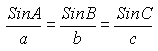From Jon, a secondary school student: What would the equation be to determine the height of an object if you cannot aproach the base of the object to use simple triangulation (taking 2 angle measurments and measuring the distance in between the two measured places, excluding eye height)? Or, more simply: How do you figure out the length of all sides of a scalene triangle if given the measure of all angles and one side? Hi Jon. The Law of Sines will help you out. If you know four of the six lengths and angles of a triangle, you can calculate the other two:Hope this helps! Stephen La Rocque.